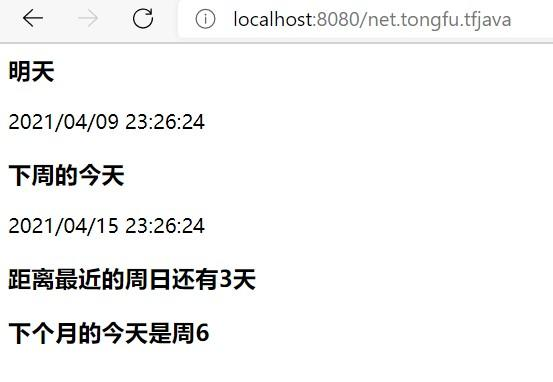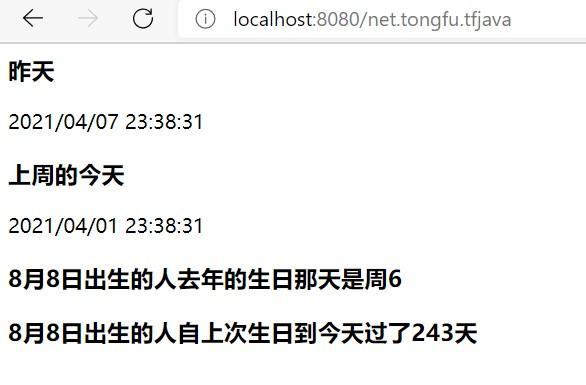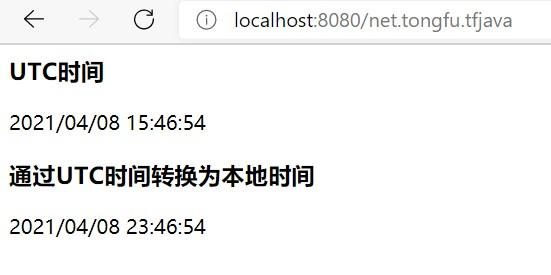# 当前时间

## UTC时间

```SimpleDateFormat simpleDateFormat = new SimpleDateFormat("yyyy/MM/dd HH:mm:ss");
simpleDateFormat.setTimeZone(TimeZone.getTimeZone("UTC"));

out += "<h3>当前UTC时间</h3>";
out += simpleDateFormat.format(Calendar.getInstance().getTime());```

## 本地时间

```SimpleDateFormat simpleDateFormat = new SimpleDateFormat("yyyy/MM/dd HH:mm:ss");

out += "<h3>本地时间</h3>";
out += simpleDateFormat.format(Calendar.getInstance().getTime());```

# 创建时间

Calendar对象的set方法可以顺序传入年、月、日、时、分、秒的定制参数，用来构造一个特定时间。需要注意的是月份参数是从0开始计算的，也就是说“1”代表2月，“0”才是1月。

```SimpleDateFormat simpleDateFormat = new SimpleDateFormat("yyyy/MM/dd HH:mm:ss");

out += "<h3>创建时间</h3>";
Calendar calendar = Calendar.getInstance();
calendar.set(2021, 3, 8, 21, 56, 38);
out += simpleDateFormat.format(calendar.getTime());```

# 时间偏移

## 偏移单位

Calendar对象的add方法的偏移单位有很多，福哥摘抄了一部分给大家参考。

• 1：年

• 2：月

• 3：周

• 5：天

• 11：时

• 12：分

• 13：秒

• 14：微秒

## 未来

```SimpleDateFormat simpleDateFormat = new SimpleDateFormat("yyyy/MM/dd HH:mm:ss");
Calendar calendar;

out += "<h3>明天</h3>";
calendar = Calendar.getInstance();
calendar.add(5, 1);
out += simpleDateFormat.format(calendar.getTime());
out += "<h3>下周的今天</h3>";
calendar = Calendar.getInstance();
calendar.add(5, 7);
out += simpleDateFormat.format(calendar.getTime());

simpleDateFormat = new SimpleDateFormat("u");
calendar = Calendar.getInstance();
out += "<h3>距离最近的周日还有" + String.valueOf(7-Integer.parseInt(simpleDateFormat.format(calendar.getTime()))) + "天</h3>";
simpleDateFormat = new SimpleDateFormat("u");
calendar = Calendar.getInstance();
calendar.add(5, 30);
out += "<h3>下个月的今天是周" + simpleDateFormat.format(calendar.getTime()) + "</h3>";```## 过去

```SimpleDateFormat simpleDateFormat = new SimpleDateFormat("yyyy/MM/dd HH:mm:ss");
Calendar calendar;

out += "<h3>昨天</h3>";
calendar = Calendar.getInstance();
calendar.add(5, -1);
out += simpleDateFormat.format(calendar.getTime());
out += "<h3>上周的今天</h3>";
calendar = Calendar.getInstance();
calendar.add(5, -7);
out += simpleDateFormat.format(calendar.getTime());

simpleDateFormat = new SimpleDateFormat("u");
calendar = Calendar.getInstance();
calendar.set(2021, 7, 8, 0, 0, 0);
calendar.add(1, -1);
out += "<h3>8月8日出生的人去年的生日那天是周" + simpleDateFormat.format(calendar.getTime()) + "</h3>";
simpleDateFormat = new SimpleDateFormat("u");
calendar = Calendar.getInstance();
calendar.set(2021, 7, 8, 0, 0, 0);
calendar.add(1, -1);
out += "<h3>8月8日出生的人自上次生日到今天过了" + Long.valueOf((Calendar.getInstance().getTime().getTime()-calendar.getTime().getTime())/(3600*24*1000)) + "天</h3>";```# 格式化

## 格式

• G：Era designator

• y：Year

• Y：Week year

• M：Month in year

• w：Week in year

• W：Week in month

• D：Day in year

• d：Day in month

• F：Day of week in month

• E：Day name in week

• u：Day number of week (1 = Monday, ..., 7 = Sunday)

• a：Am/pm marker

• H：Hour in day (0-23)

• k：Hour in day (1-24)

• K：Hour in am/pm (0-11)

• h：Hour in am/pm (1-12)

• m：Minute in hour

• s：Second in minute

• S：Millisecond

• z：Time zone

• Z：Time zone

• X：Time zone

# UTC时间转本地时间

## 时区转换

```SimpleDateFormat simpleDateFormat = new SimpleDateFormat("yyyy/MM/dd HH:mm:ss");
simpleDateFormat.setTimeZone(TimeZone.getTimeZone("UTC"));
String nowUTC;

out += "<h3>UTC时间</h3>";
nowUTC = simpleDateFormat.format(Calendar.getInstance().getTime());
out += nowUTC;
out += "<h3>通过UTC时间转换为本地时间</h3>";
try {
Date dateNowUTC = simpleDateFormat.parse(nowUTC);
simpleDateFormat.setTimeZone(TimeZone.getDefault());
out += simpleDateFormat.format(dateNowUTC);
}
catch (ParseException e){
e.printStackTrace();
}```# 格式转换

Java有几种时间的对象，包括Calendar、Date、Timestamp以及字符串类型。这几种时间的对象之间是如何转换的呢？

## Calendar -> Date

`Date date = calendar.getTime();`

## Date -> Timestamp

`Timestamp timestamp = new Timestamp(date.getTime());`

## Timestamp -> String

```SimpleDateFormat simpleDateFormat = new SimpleDateFormat("yyyy-MM-dd HH:mm:ss");
String datetime = simpleDateFormat.format(timestamp);```

## String -> Calendar

```try {
date = simpleDateFormat.parse(datetime);
calendar.setTime(date);
}
catch (ParseException e){
e.printStackTrace();
}```